# Core couplings - Ideal Transformer

This section describes Ideal Transformer core coupling, including single-phase coupling component, three-phase coupling component, four-phase coupling component and five-phase coupling component

Core coupling components are used to divide the circuit into sub circuits that will be simulated in separate cores of a single HIL device. In addition, the ideal transformer block of the core coupling introduces fixed time delay that is equal to one simulation step ( 0.5 µs, 1 µs or 2 µs ...) between measured variables and corresponding controlled sources, which is negligible for most practical systems. There are four core coupling in the library: single phase, three phase, four and five phase device couplings.

Depending on the position, core coupling elements can introduce instabilities in the circuit. In order to verify the stability of core coupling components a coupling stability analysis tool is available. It is described in Figure 3.

For more details about coupling placement please refer to Coupling component placement and parametrization - Ideal Transformer based couplings.

When coupling components are added to the circuit topological conflicts can occur (described in Topological Conflicts). These conflicts are solved by adding snubber circuits in parallel with coupling's current source and/or in series with coupling's voltage source. Snubbers are embedded in coupling components. If parameter Fixed snubber is set to False, than snubbers used only when there are topological issues. If parameter Fixed snubber is set to True, the chosen snubbers will always be in the circuit.

There are three options for snubbers for both, voltage and current source side:

1. without snubber - "none"
2. resistive - "R1" and "R2"
3. first order filter - "R1-C1" (resistance in series with capacitor)- "R2||L1"(resistance in parallel with an inductor)

On Figure 2 a coupling building block with and without snubbers is shown.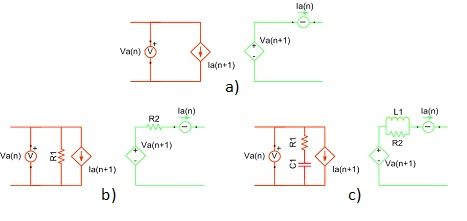Figure 2. Basic building block of a coupling component: a) ideal transformer, without snubbers; b) ideal transformer with resistive snubbers; c) ideal transformer with RC/RL snubbers

For core coupling components, coupling stability analysis routine is available. Coupling stability analysis checks the stability of all core coupling components in the model. This option is disabled by default. It can be enabled in Schematic settings -> Circuit Solver settings -> Enable coupling stability analysis as shown in figure below.

If core coupling stability analysis is enabled, than during the compilation, a stability report is printed for all couplings in the circuit. For each coupling, there can be three massages: coupling element is stable, coupling element is unstable and coupling element is around stability border. For the first two cases, the message is clear. The third case, around stability border, means that the coupling might be ether stable or unstable, it is not possible to exactly determine since it is working close to the stability border. In this case, it is recommended to make it more stable.

Coupling stability routine analysis the stability through all the switch permutations present in the model. For certain switch permutations, coupling elements can be stable while for other permutations it can be unstable. If there is only one switch permutation that makes the coupling component unstable, the report will report that the component is unstable without further details. As an example, there is a simple circuit containing one switch, voltage sources, voltage measurements, resistors and a core coupling component shown in figure below. The stability analysis reports unstable coupling. The switch has two positions, open and close. If the switch is closed than the coupling is stable, but if it is open, the coupling is unstable. Permutations of the circuit are shown in figure below. This means that even if a coupling is determined to be unstable, the model can behave correctly for some, usually majority of the switch permutations.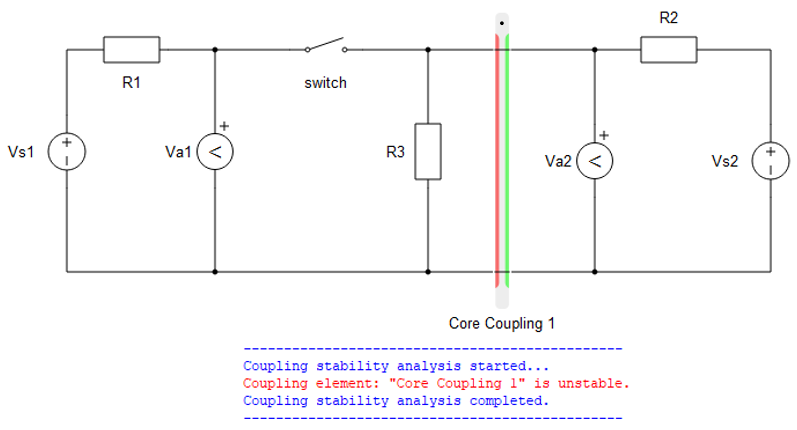Figure 5. Unstable coupling element in circuit with one switch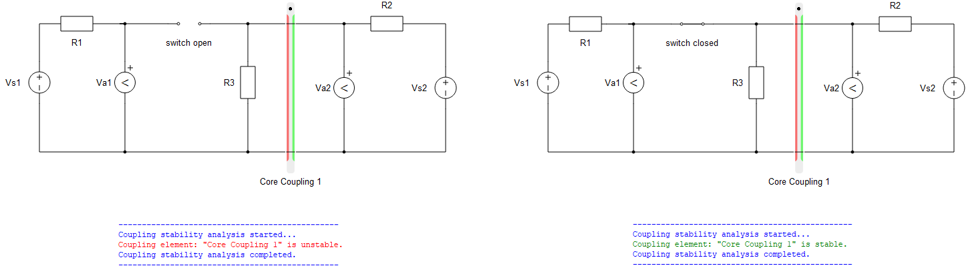Figure 6. Permutations of switch and stability analysis result of each permutation

## Single-phase coupling component

A coupling component is an ideal transformer with a transfer ratio of one, used to divide the simulated circuit into two sub-circuits. Consequently, the two sub-circuits are distributed and executed in two HIL processor cores. In addition, this ideal transformer block introduces the one time step delay (i.e. 1 µs) between measured variables and corresponding controlled sources, which is negligible for most practical systems.

Single phase coupling is constructed of one coupling component building block.

Table 1. Coupling components in the HIL Toolbox
component component dialog window component parameters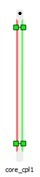Single phase coupling• Current source side snubber type
• Current source side resistance: R1
• Current source side capacitance: C1
• Voltage source side snubber type
• Voltage source side resistance: R2
• Voltage source side inductance: L1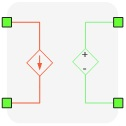Figure 7. The schematic block diagram of a single phase coupling component

An example of coupling component usage is to split a back-to-back inverter rectifier circuit along the DC-link capacitor. Since the voltage on the capacitor cannot change instantaneously, the delay between measuring DC-link voltage and re-playing it on the secondary side of the coupling component can be easily neglected.

For more details about coupling placement please refer to Coupling component placement and parametrization - Ideal Transformer based couplings.

## Three-phase coupling component

A three-phase coupling component consists of two ideal transformers with a transfer ratio of one, used to divide the circuit simulated into sub-circuits, along the three-phase connection. Consequently, sub-circuits are distributed and executed in two HIL processor cores. In addition, this ideal transformer block introduces the one time step delay (i.e. 1 µs) between measured variables and corresponding controlled sources, which is negligible for most practical systems.

Table 2. Coupling components in the HIL Toolbox
component component dialog window component parameters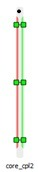Three phase coupling• Current source side snubber type
• Current source side resistance: R1
• Current source side capacitance: C1
• Voltage source side snubber type
• Voltage source side resistance: R2
• Voltage source side inductance: L1

Three phase coupling component is constructed of two coupling component building blocks connected in star connection as shown in Figure 8.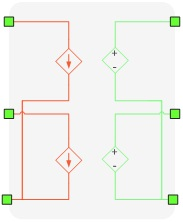Figure 8. The schematic block diagram of a three-phase coupling component - star connection of two single phase couplings

An example of three phase coupling component usage is to split a back-to-back three level NPC inverter-rectifier circuit along the DC-link capacitors. Since the voltage on the capacitors cannot change instantaneously, the delay between measuring DC-link voltage and re-playing it on the secondary side of the coupling component can be easily neglected.

For more details about coupling placement please refer to Coupling component placement and parametrization - Ideal Transformer based couplings.

## Four-phase coupling component

A four-phase coupling component consists of three ideal transformers with a transfer ratio of one, used to divide the simulated circuit into sub-circuits. Consequently, sub-circuits are distributed and executed in two HIL processor cores. In addition, this ideal transformer block introduces the one time step delay (i.e. 1 µs) between measured variables and corresponding controlled sources, which is negligible for most practical systems.

Table 3. Coupling components in the HIL Toolbox
component component dialog window component parameters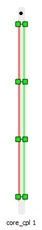Four phase coupling• Current source side snubber type
• Current source side resistance: R1
• Current source side capacitance: C1
• Voltage source side snubber type
• Voltage source side resistance: R2
• Voltage source side inductance: L1

Four phase coupling component is constructed of three coupling component building blocks connected in star connection as shown in Figure 9.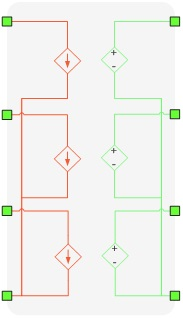Figure 9. The schematic block diagram of a four-phase coupling component - star connection of three single phase couplings

For more details about coupling placement please refer to Coupling component placement and parametrization - Ideal Transformer based couplings.

## Five-phase coupling component

A five-phase coupling component consists of four ideal transformers with a transfer ratio of one, used to divide the simulated circuit into sub-circuits. Consequently, sub-circuits are distributed and executed in two HIL processor cores. In addition, this ideal transformer block introduces the one time step delay (i.e. 1 µs) between measured variables and corresponding controlled sources, which is negligible for most practical systems.

Table 4. Coupling components in the HIL Toolbox
component component dialog window component parameters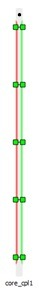Five phase coupling• Current source side snubber type
• Current source side resistance: R1
• Current source side capacitance: C1
• Voltage source side snubber type
• Voltage source side resistance: R2
• Voltage source side inductance: L1

Five phase coupling component is constructed of three coupling component building blocks connected in star connection as shown in Figure 10.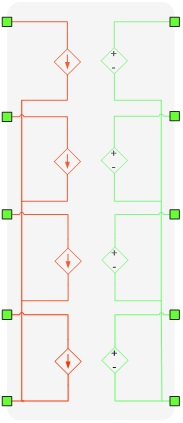Figure 10. The schematic block diagram of a five-phase coupling component - star connection of three single phase couplings

For more details about coupling placement please refer to Coupling component placement and parametrization - Ideal Transformer based couplings.